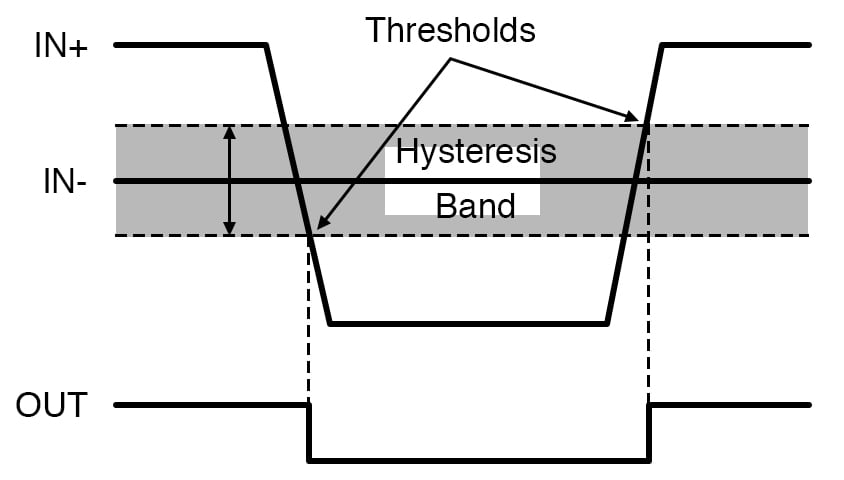# The Op-Amp Voltage Comparator Circuit

January 29, 2020 by Robert Keim
In this video we will discuss circuits that use an operational amplifier to compare the amplitude of one signal to the amplitude of another signal or to a reference voltage. These analog comparator circuits can produce output signals that are compatible with digital components.
• Share

An operational amplifier is a high-gain amplifier with a differential input stage. However, most op-amp circuits do not have high gain and do not perform differential amplification. Rather, we use negative feedback to turn a high-gain differential amplifier into a high-performance and user-friendly circuit that amplifies individual ground-referenced signals.

## The Basic Comparator

The only common op-amp application that uses the device in its original high-gain, differential-input configuration is the voltage comparator. The purpose of a voltage comparator is to generate an output signal that corresponds to the greater-than-or-less-than relationship between the voltages at the op-amp’s input terminals. If we think of the comparator’s output as a digital signal, we can say that the comparator generates a logic high when the voltage at the non-inverting input is higher than the voltage at the inverting input and a logic low when the voltage at the non-inverting input is lower than the voltage at the inverting input.

Comparator operation occurs as a natural result of the op-amp’s extremely high gain. As shown in the diagram below, the full gain (AOL) is applied to the difference between the voltage at the non-inverting input terminal and the voltage at the inverting input terminal.$V_{OUT} = A_{OL} \times (V_{IN+} - V_{IN-}) = A_{OL} \times V_{DIFF}$

Thus, even a very small positive VDIFF will cause the op-amp’s output to saturate at or near the positive supply voltage, and even a very small negative VDIFF will cause the output to saturate at or near the negative supply voltage. If the negative supply is connected to ground, the comparator produces a digital signal that varies between logic low (≈0 V) and logic high (≈VCC, which could be 3.3 V or 5 V).

Though op-amps can be used as comparators, it’s important to understand that typical op-amps are not optimized for this type of functionality—open-loop, rail-to-rail saturation is very different from negative-feedback-based signal amplification. If you want to improve the performance of your comparator circuit, you can use an amplifier IC that is sold specifically as a comparator.

## Comparator with Reference Voltage

A common comparator application is producing an output signal that indicates whether an input signal is above or below a specified threshold voltage. For example, you might want to manually deactivate one of the components on a circuit board when its supply voltage drops below 3 V.

These types of comparator tasks are accomplished by generating a reference voltage and using this voltage as one of the inputs to the comparator. If you need an extremely precise threshold, you can use a voltage-reference IC, but in many cases a resistive divider is adequate.## Comparator with Hysteresis

The open-loop comparator topology discussed thus far has a serious limitation: noise will cause the op-amp to produce spurious output transitions when VDIFF is near 0 V.##### When a comparator has only one threshold, noise (represented by the low-amplitude fluctuations in the green trace) can cause spurious output transitions (as seen in the behavior of the red trace).

For example, let’s say that a microcontroller needs to execute a block of code each time a periodic sensor signal exceeds a threshold voltage. We will use a comparator to generate a signal that initiates the execution of this code block. However, when the sensor signal approaches the threshold, small-amplitude, high-frequency variations can cause the signal to quickly move above and below the threshold. This causes VDIFF to vary between negative values and positive values, and this in turn leads to multiple output transitions. These transitions are undesirable because they represent noise behavior rather than the behavior of the “authentic” input signal.

The problem of spurious output transitions is resolved by incorporating hysteresis into the comparator circuit. The term “hysteresis” refers to the technique of using different thresholds for negative-to-positive transitions and positive-to-negative transitions. This creates a hysteresis band that extends above and below VDIFF = 0 V.As shown in the following diagram, we can create different negative-to-positive and positive-to-negative thresholds by incorporating positive feedback.## Summary

• A comparator generates an output signal that indicates which of two input signals has the higher voltage.
• An op-amp can function as a comparator because it combines a differential input stage with very high gain.
• A voltage-reference IC or a resistive divider can be used to generate a threshold voltage for comparator circuits.
• Spurious output transitions can be suppressed by using positive feedback to create a hysteresis band.